• 累计撰写 270 篇文章
• 累计创建 6 个标签
• 累计收到 1 条评论

### 目 录CONTENT# STL中的空间配置器.md2021-08-22 / 0 评论 / 0 点赞 / 8 阅读 / 8,343 字 / 正在检测是否收录...

# STL中的空间配置器

@[toc]

## 2. 为什么需要空间配置器

•  空间申请与释放需要用户自己管理，容易造成内存泄漏
•  频繁向系统申请小块内存块，容易造成内存碎片
•  频繁向系统申请小块内存，影响程序运行效率
•  直接使用malloc与new进行申请，每块空间前有额外空间浪费
•  申请空间失败怎么应对
•  代码结构比较混乱，代码复用率不高
•  未考虑线程安全问题

## 3. SGI-STL空间配置器实现原理

•  以上提到的几点不足之处，最主要还是：频繁向系统申请小块内存造成的
•  那什么才算是小块内存？SGI-STL 以128作为小块内存与大块内存的分界线
•  将空间配置器其分为两级结构，一级空间配置器处理大块内存， 二级空间配置器处理小块内存。

### 3.1 一级空间配置器

template <int inst>
class __malloc_alloc_template
{
private:
static void *oom_malloc(size_t);

public:

// 对malloc的封装
static void * allocate(size_t n)
{
// 申请空间成功，直接返回，失败交由oom_malloc处理
void *result = malloc(n);
if (0 == result)
result = oom_malloc(n);
return result;
}

// 对free的封装
static void deallocate(void *p, size_t /* n */)
{ free(p);}

// 模拟set_new_handle
// 该函数的参数为函数指针，返回值类型也为函数指针
// void (* set_malloc_handler( void (*f)() ) )()
static void (* set_malloc_handler(void (*f)()))()
{
void (* old)() = __malloc_alloc_oom_handler;
__malloc_alloc_oom_handler = f;
return(old);
}
};

// malloc申请空间失败时代用该函数
template <int inst>
void * __malloc_alloc_template<inst>::oom_malloc(size_t n)
{
void (* my_malloc_handler)();
void *result;
for (;;)
{
// 检测用户是否设置空间不足应对措施，如果没有设置，抛异常，模式new的方式
my_malloc_handler = __malloc_alloc_oom_handler;
if (0 == my_malloc_handler)
{
}

// 如果设置，执行用户提供的空间不足应对措施
(*my_malloc_handler)();
// 继续申请空间，可能就会申请成功
result = malloc(n);
if (result)
return(result);
}
}
typedef __malloc_alloc_template<0> malloc_alloc;


### 3.2 二级空间配置器

二级空间配置器专门负责处理小于128字节的小块内存。如何才能提升小块内存的申请与释放的方式呢？SGI- STL采用了内存池的技术来提高申请空间的速度以及减少额外空间的浪费，采用哈希桶的方式来提高用户获 取空间的速度与高效管理

#### 3.1 内存池

•  内存池就是：先申请一块比较大的内存块已做备用，当需要内存时，直接到内存池中去去，当池中空间不够时，再向内存中去取，当用户不用时，直接还回内存池即可。
•  避免了频繁向系统申请小块内存所造成的效率低、内存碎片以及额外浪费的问题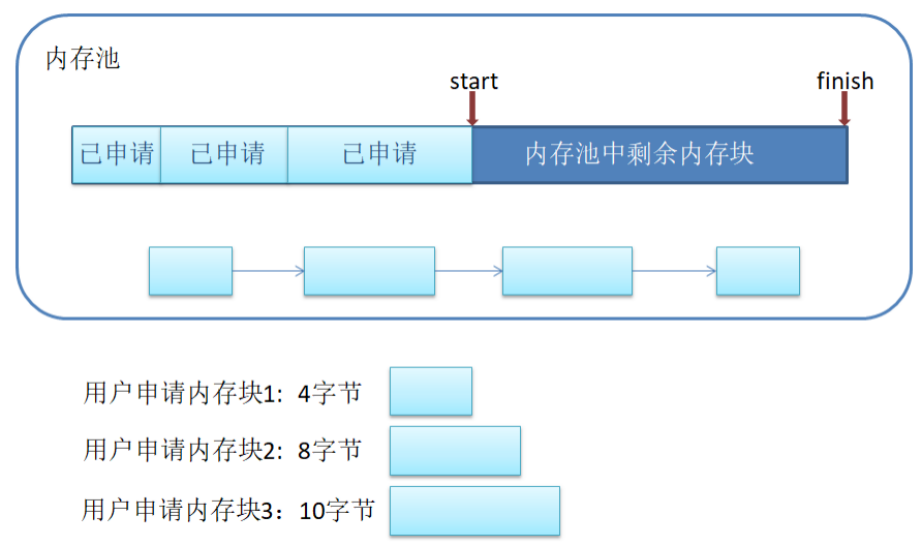#### 3.2 SGI-STL中二级空间配置器设计

•  SGI-STL中的二级空间配置器使用了内存池技术，但没有采用链表的方式对用户已经归还的空间进行管理(因为用户申请空间时在查找合适的小块内存时效率比较低)
•  而是采用了哈希桶的方式进行管理
•  那是否需要 128桶个空间来管理用户已经归还的内存块呢？答案是不需要，因为用户申请的空间基本都是4的整数倍，其 他大小的空间几乎很少用到。因此：SGI-STL将用户申请的内存块向上对齐到了8的整数倍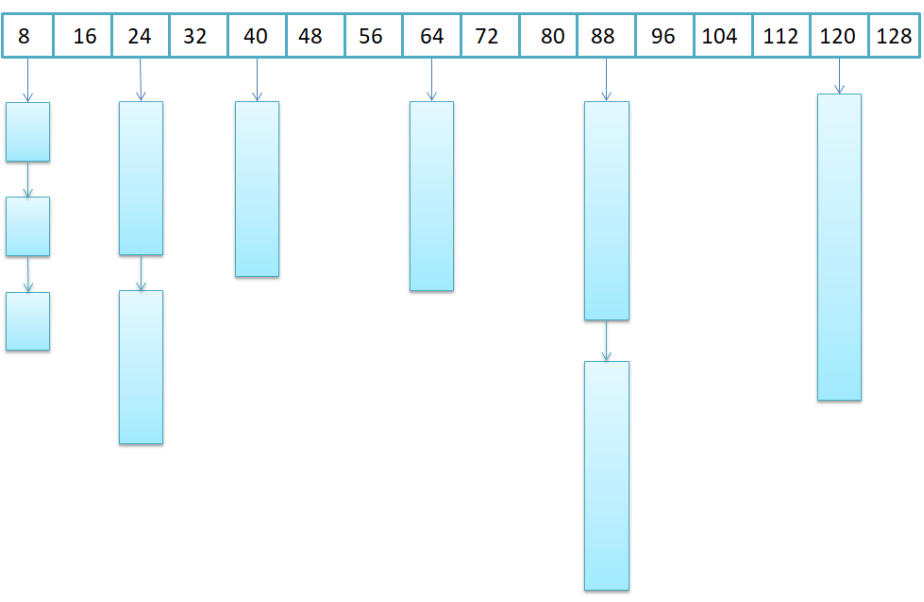#### 3.3 SGI-STL二级空间配置器之空间申请

1. 前期的准备

// 去掉代码中繁琐的部分
template <int inst>
class __default_alloc_template
{
private:
enum {__ALIGN = 8}; // 如果用户所需内存不是8的整数倍，向上对齐到8的整数倍
enum {__MAX_BYTES = 128}; // 大小内存块的分界线
enum {__NFREELISTS = __MAX_BYTES/__ALIGN}; // 采用哈希桶保存小块内存时所需桶的个数
// 如果用户所需内存块不是8的整数倍，向上对齐到8的整数倍
static size_t ROUND_UP(size_t bytes)
{
return (((bytes) + __ALIGN-1) & ~(__ALIGN - 1));
}
private:
// 用联合体来维护链表结构
union obj
{
char client_data; /* The client sees this. */
};
private:
static obj * free_list[__NFREELISTS];
// 哈希函数，根据用户提供字节数找到对应的桶号
static size_t FREELIST_INDEX(size_t bytes)
{
return (((bytes) + __ALIGN-1)/__ALIGN - 1);
}
// start_free与end_free用来标记内存池中大块内存的起始与末尾位置
static char *start_free;
static char *end_free;
// 用来记录该空间配置器已经想系统索要了多少的内存块
static size_t heap_size;
// ...
} ;


2. 申请空间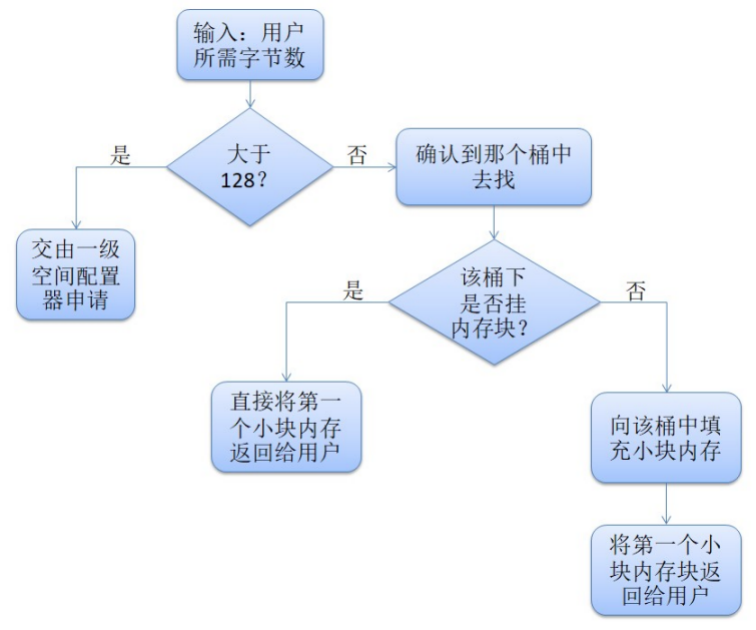// 函数功能：向空间配置器索要空间
// 参数n: 用户所需空间字节数
// 返回值：返回空间的首地址
static void * allocate(size_t n)
{
obj * __VOLATILE * my_free_list;
obj * __RESTRICT result;
// 检测用户所需空间释放超过128(即是否为小块内存)
if (n > (size_t) __MAX_BYTES)
{
// 不是小块内存交由一级空间配置器处理
return (malloc_alloc::allocate(n));
}
// 根据用户所需字节找到对应的桶号
my_free_list = free_list + FREELIST_INDEX(n);
result = *my_free_list;
// 如果该桶中没有内存块时，向该桶中补充空间
if (result == 0)
{
// 将n向上对齐到8的整数被，保证向桶中补充内存块时，内存块一定是8的整数倍
void *r = refill(ROUND_UP(n));
return r;
}
// 维护桶中剩余内存块的链式关系
return (result);
};


3. 填充内存块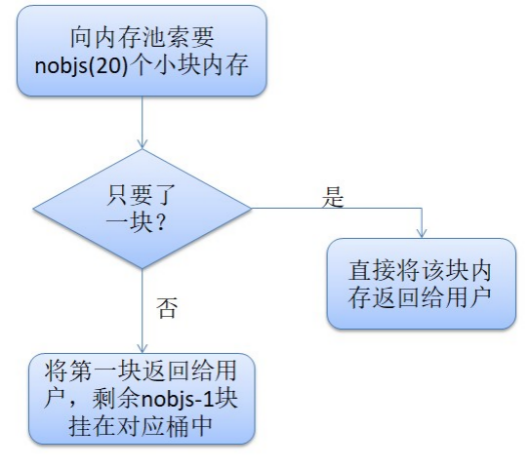// 函数功能：向哈希桶中补充空间
// 参数n：小块内存字节数
// 返回值：首个小块内存的首地址
template <int inst>
void* __default_alloc_template<inst>::refill(size_t n)
{
// 一次性向内存池索要20个n字节的小块内存
int nobjs = 20;
char * chunk = chunk_alloc(n, nobjs);
obj ** my_free_list;
obj *result;
obj *current_obj, *next_obj;
int i;
// 如果只要了一块，直接返回给用户使用
if (1 == nobjs)
return(chunk);
// 找到对应的桶号
my_free_list = free_list + FREELIST_INDEX(n);
// 将第一块返回值用户，其他块连接在对应的桶中
// 注：此处代码逻辑比较简单，但标准库实现稍微有点复杂，同学们可以自己实现
result = (obj *)chunk;
*my_free_list = next_obj = (obj *)(chunk + n);
for (i = 1; ; i++)
{
current_obj = next_obj;
next_obj = (obj *)((char *)next_obj + n);
if (nobjs - 1 == i)
{
break;
}
else
{
}
}
return(result);
}


4. 向内存池中索要空间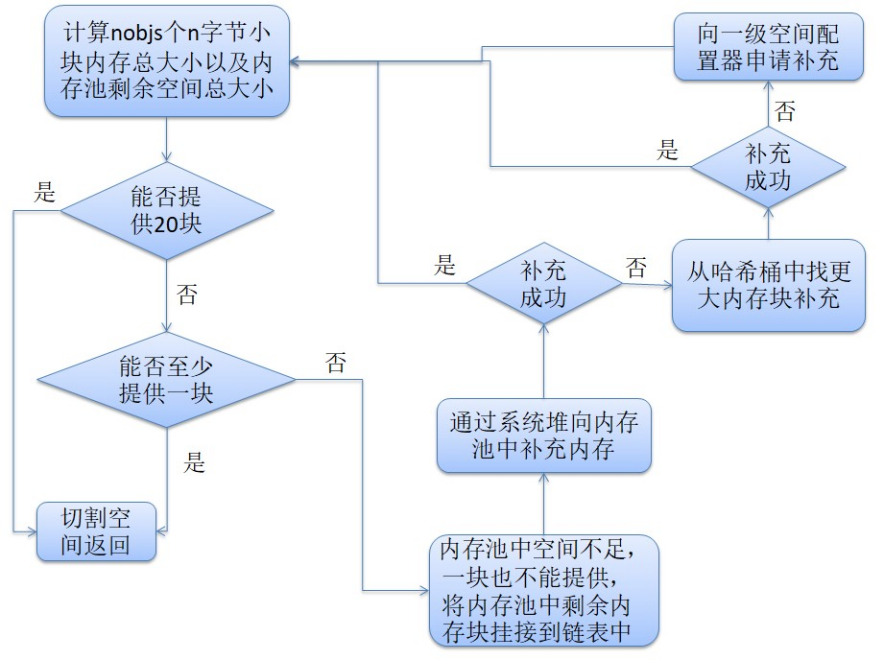template <int inst>
char* __default_alloc_template<inst>::chunk_alloc(size_t size, int& nobjs)
{
// 计算nobjs个size字节内存块的总大小以及内存池中剩余空间总大小
char * result;
size_t total_bytes = size * nobjs;
size_t bytes_left = end_free - start_free;
// 如果内存池可以提供total_bytes字节，返回
if (bytes_left >= total_bytes)
{
result = start_free;
start_free += total_bytes;
return(result);
}
else if (bytes_left >= size)
{
// nobjs块无法提供，但是至少可以提供1块size字节内存块，提供后返回
nobjs = bytes_left/size;
total_bytes = size * nobjs;
result = start_free;
start_free += total_bytes;
return(result);
}
else
{
// 内存池空间不足，连一块小块村内都不能提供
// 向系统堆求助，往内存池中补充空间
// 计算向内存中补充空间大小：本次空间总大小两倍 + 向系统申请总大小/16
size_t bytes_to_get = 2 * total_bytes + ROUND_UP(heap_size >> 4);
// 如果内存池有剩余空间(该空间一定是8的整数倍)，将该空间挂到对应哈希桶中
if (bytes_left > 0)
{
// 找对用哈希桶，将剩余空间挂在其上
obj ** my_free_list = free_list + FREELIST_INDEX(bytes_left);
((obj *)start_free) -> free_list_link = *my_free_list;
*my_ree_list = (obj *)start_free;
}

// 通过系统堆向内存池补充空间，如果补充成功，递归继续分配
start_free = (char *)malloc(bytes_to_get);
if (0 == start_free)
{
// 通过系统堆补充空间失败，在哈希桶中找是否有没有使用的较大的内存块
int i;
obj ** my_free_list, *p;
for (i = size; i <= __MAX_BYTES; i += __ALIGN)
{
my_free_list = free_list + FREELIST_INDEX(i);
p = *my_free_list;
// 如果有，将该内存块补充进内存池，递归继续分配
if (0 != p)
{
start_free = (char *)p;
end_free = start_free + i;
return(chunk_alloc(size, nobjs));
}
}
// 山穷水尽，只能向一级空间配置器求助
// 注意：此处一定要将end_free置空，因为一级空间配置器一旦抛异常就会出问题
end_free = 0;
start_free = (char *)malloc_alloc::allocate(bytes_to_get);
}
// 通过系统堆向内存池补充空间成功，更新信息并继续分配
heap_size += bytes_to_get;
end_free = start_free + bytes_to_get;
return(chunk_alloc(size, nobjs));
}
}


3.4 SGI-STL二级空间配置器之空间回收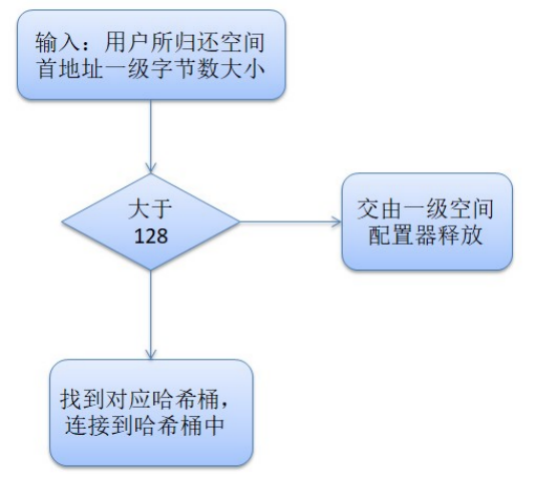// 函数功能：用户将空间归还给空间配置器
// 参数：p空间首地址 n空间总大小
static void deallocate(void *p, size_t n)
{
obj *q = (obj *)p;
obj ** my_free_list;
// 如果空间不是小块内存，交给一级空间配置器回收
if (n > (size_t) __MAX_BYTES)
{
malloc_alloc::deallocate(p, n);
return;
}
// 找到对应的哈希桶，将内存挂在哈希桶中
my_free_list = free_list + FREELIST_INDEX(n);
*my_free_list = q;
}


### 3.3 空间配置器的默认选择

SGI-STL默认使用一级还是二级空间配置器，通过USE_MALLOC宏进行控制：

#ifdef __USE_MALLOC
typedef malloc_alloc alloc;
typedef malloc_alloc single_client_alloc;
#else
// 二级空间配置器定义
#endif


### 3.4 空间配置器的再次封装

// T: 元素类型
// Alloc: 空间配置器
// 注意：该类只负责申请与归还对象的空间，不否则空间中对象的构造与析构
template<class T, class Alloc>
class simple_alloc
{
public:
// 申请n个T类型对象大小的空间
static T *allocate(size_t n)
{
return 0 == n? 0 : (T*) Alloc::allocate(n * sizeof (T));
}
// 申请一个T类型对象大小的空间
static T *allocate(void)
{
return (T*) Alloc::allocate(sizeof (T));
}
// 释放n个T类型对象大小的空间
static void deallocate(T *p, size_t n)
{
if (0 != n)
Alloc::deallocate(p, n * sizeof (T));
}
// 释放一个T类型对象大小的空间
static void deallocate(T *p)
{
Alloc::deallocate(p, sizeof (T));
}
};


### 3.5 对象的构造与释放

// 归还空间时，先先调用该函数将对象中资源清理掉
template <class T>
inline void destroy(T* pointer)
{
pointer->~T();
}
// 空间申请好后调用该函数：利用placement-new完成对象的构造
template <class T1, class T2>
inline void construct(T1* p, const T2& value)
{
new (p) T1(value);
}


1. 在释放对象时，需要根据对象的类型确定是否调用析构函数(类型萃取)
2. 对象的类型可以通过迭代器获萃取到

## 4. 与容器结合

template <class T, class Alloc = alloc>
class list
{
// ...
// 实例化空间配置器
typedef simple_alloc<list_node, Alloc> list_node_allocator;
// ...
protected:
{
// 调用空间配置器接口先申请节点的空间
return list_node_allocator::allocate();
}
// 将节点归还给空间配置器
{
list_node_allocator::deallocate(p);
}
// 创建节点：1. 申请空间 2. 完成节点构造
{
construct(&p->data, x);
return p;
}
// 销毁节点： 1. 调用析构函数清理节点中资源 2. 将节点空间归还给空间配置器
{
destroy(&p->data);
put_node(p);
}
// ...
iterator insert(iterator position, const T& x)
{
tmp->next = position.node;
tmp->prev = position.node->prev;
position.node->prev = tmp;
return tmp;
}
iterator erase(iterator position)
{
prev_node->next = next_node;
next_node->prev = prev_node;
destroy_node(position.node);
return iterator(next_node);
}
// ...
};


## 5.总结

•  new运算分两个阶段：

(1)调用::operator new配置内存;
(2)调用对象构造函数构造对象内容

•  delete运算分两个阶段：

(1)调用对象希构函数；
(2)调用operator delete释放内存

•  为了精密分工，STL allocator将两个阶段操作区分开来：
•  内存配置有alloc::allocate()负责，内存释放由alloc::deallocate()负责；
•  对象构造由::construct()负责，对象析构由::destroy()负责。

•  SGI STL采用了两级配置器，当分配的空间大小超过128字节时，会使用第一级空间配置器；
•  当分配的空间大小小于128字节时，将使用第二级空间配置器。
•  第一级空间配置器直接使用malloc()、realloc()、free()函数进行内存空间的分配和释放
•  而第二级空间配置器采用了内存池技术，通过空闲链表来管理内存。
0